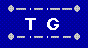Solving Diophantine Equations Home  | Send Your Feedback

Diophantine Equations are those in which there are more
variables than equations and hence multiple solutions.
Usually, integer solutions are required.

Diophantine Equations of the form
• A x + B y = C
where A, B, and C are given.

Since A(x + n*B) + B(y - n*A) = Ax + By + ABn - ABn,
given one solution, you can add or subtract any multiple of B to x, while doing the opposite (subtracting or adding) with the same multiple of A to y.

Example: "Solve 7x + 13y = 102"

Starting from x = 9, y = 3 (which gives 63 + 39 = 102)
other solutions are:
 N 9 + N*13 3 - N*7 7 x + 13 y = 102 -2 9-26 = -17 3+14=17 7*(-17) + 13*17 = -119 + 221 = 102 -1 9-13 = -4 3+7=10 7*(-4) + 13*10 = -28 + 130 =102 1 9+13 = 22 3-7=-4 7*22 - 13*4 = 154-52 = 102 2 9+26 = 35 3-14=-11 7*35 - 13*11 = 245-143 = 102

To solve a Diophantine Equation,
enter the three integers you want as coefficients,
and press "Solve".

 Ax + By = C A = * x + B = * y = C =

The Old Water Jug Problem
explains the solution method used.